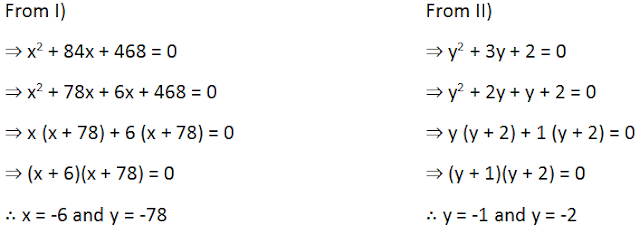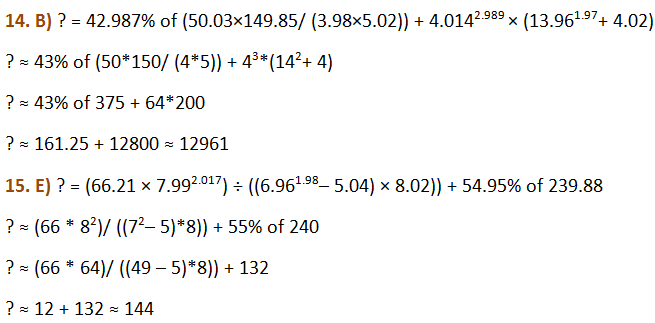# Quantitative Aptitude Practice Questions for IBPS Clerk – Set 7

Quantitative Aptitude Practice Questions for IBPS Clerk – Set 7
Directions (1 – 5): In the following question, one or two equation(s) is/are given. You have to solve both the equations and find the relation between ‘x’ and ‘y’ and mark correct answer.
(a)  x > y
(b)  x ≥ y
(c)  x< y
(d)  x ≤ y
(e)  x = y or the relation cannot be determinedDirections (6 – 10): What should come in place of question mark (?) in the following number series?
6. 7, 35, 105, 525, 1575, 7875, ?
(a)  39375
(b)  23625
(c)  11815
(d)  15750
(e)  None of these
7. 5120    1280    320    80    ?
(a)  16
(b)  24
(c)  20
(d)  40
(e)  None of these
8. 117      389      525      593      627      (?)
(a)  654
(b)  640
(c)  634
(d)  630
(e)  None of these
9. 16,       4,         2,         1.5,      (?),        1.875
(a)  4
(b)  1.875
(c)  1.5
(d)  2
(e)  1.75
10. 2,         6,         14,       30,       (?),     126
(a)  62
(b)  98
(c)  87
(d)  113
(e)  97
Directions (11 – 15): What approximate value will come in place of question mark (?) in the following question? (Note: – You are not expected to calculate the exact value)
11. 455.45 + 289.89 + 921.9 = 678.78 – ?
(a) -988
(b) -862
(c) -781
(d) -908
(e) -912
12. 74.68% of (24.77 × 7.89) + 665.59 =? – 110.91
(a) 821
(b) 754
(c) 927
(d) 776
(e) 910
13. (449.95 × 199.99 × 4501.23) ÷ (69.78 × 90.9 × 1449.85) = ?
(a) 21
(b) 86
(c) 52
(d) 44
(e) 79Solutions:
1. E) We will solve both the equations separately.Comparing the values of x and y, we get,
For x = -4, x ≤ y
But for x = - 2, x > y, so relationship cannot be determined
2. A) As per the given data,When x = -6 and y = -1, then x < y
x = -6 and y = -2, then x < y
x = -78 and y = -1, then x < y
x = -78 and y = -2, then x < y
From the above, we can say x < y
3. C) As per the given data,When x = -3/2 and y = 4, then x < y
x = -3/2 and y = 3, then x < y
x = -1 and y = 4, then x < y
x = -1 and y = 3, then x < y
From the above, we can say x < y
4. A) As per the given data,x > y
5. A) As per the given data,When x = 3 and y = -4, then x > y
x = 3 and y = -8, then x > y
x = 4 and y = -4, then x > y
x = 4 and y = -8, then x > y
From the above, we can say x > y
6. B) The pattern of given series is:
→ 7,
→ 35 = 7 × 5,
→ 105 = 35 × 3,
→ 525 = 105 × 5,
→ 1575 = 525 × 3,
→ 7875 = 1575 × 5,
→ ? = 7875 × 3,
→ ? = 23625
7. C) The pattern of the given series is :
→ 5120,
→ 1280 = 5120/4,
→ 320 = 1280/4,
→ 80 = 320/4,
→ ? = 80/4,
→ ? = 20
8. E) In the given series, the difference between two consecutive numbers is as follows:
→ 389 – 117 = 272
→ 525 – 389 = 136
→ 593 – 525 = 68
→ 627 – 593 = 34
Hence it can be concluded from the values of these differences that with every next term it reduces by exactly half. i.e.
→ 272/2 = 136
→ 136/2 = 68
→ 68/2 = 34
Next difference must be = 34/2 = 17
Now, let next number be X. Then,
X 627 = 17
X = 644
Hence the required term in given number series is  644.
9. C) The pattern of the given number series is as following:
→ 16,
→ 16 × 0.25 = 4,
→ 4 × 0.5 = 2,
→ 2 × 0.75 = 1.5,
→ 1.5 × 1 = 1.5,
→ 1.5 × 1.25 = 1.875
Hence, the required term in the given number series is 1.5
10. A) The pattern of the given number series is as following:
→ 2,
→ 2 × 2 + 2 = 6,
→ 6 × 2 + 2 = 14,
→ 14 × 2 + 2 = 30,
→ 30 × 2 + 2 = 62,
→ 62 × 2 + 2 = 126
Hence, the missing number in the given number series would be 62,
11. A) 455.45 + 289.89 + 921.9 = 678.78 – ?
? ≈ 679 – 455 – 290 – 922
? = – 988
12. C) 74.68% of (24.77 * 7.89) + 665.59 =? – 110.91
75% of (25 * 8) + 666 =? – 111
? = 75% of (25 * 8) + 666 + 111
? = 150 + 777 = 927
13. D) (449.95 × 199.99 × 4501.23) ÷ (69.78 × 90.9 × 1449.85) = ?
? ≈ (450*200*4500)/ (70*91*1450)
? ≈ 44